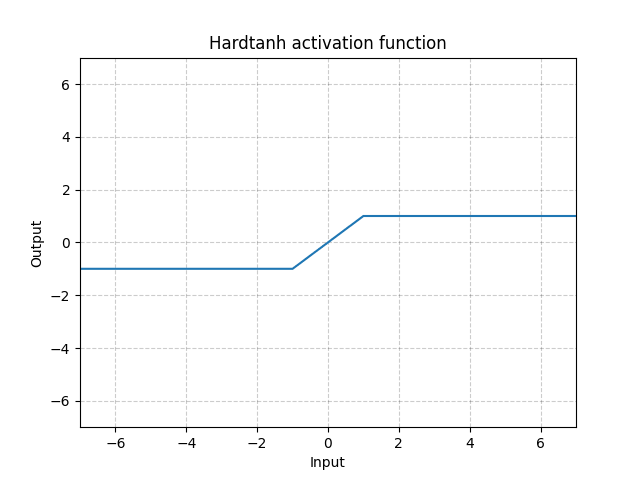/PyTorch

# Hardtanh

class torch.nn.Hardtanh(min_val: float = -1.0, max_val: float = 1.0, inplace: bool = False, min_value: Optional[float] = None, max_value: Optional[float] = None) [source]

Applies the HardTanh function element-wise

HardTanh is defined as:

$\text{HardTanh}(x) = \begin{cases} 1 & \text{ if } x > 1 \\ -1 & \text{ if } x < -1 \\ x & \text{ otherwise } \\ \end{cases}$

The range of the linear region $[-1, 1]$ can be adjusted using min_val and max_val.

Parameters
• min_val – minimum value of the linear region range. Default: -1
• max_val – maximum value of the linear region range. Default: 1
• inplace – can optionally do the operation in-place. Default: False

Keyword arguments min_value and max_value have been deprecated in favor of min_val and max_val.

Shape:
• Input: $(N, *)$ where * means, any number of additional dimensions
• Output: $(N, *)$ , same shape as the inputExamples:

>>> m = nn.Hardtanh(-2, 2)
>>> input = torch.randn(2)
>>> output = m(input)


© 2019 Torch Contributors
Licensed under the 3-clause BSD License.
https://pytorch.org/docs/1.7.0/generated/torch.nn.Hardtanh.html